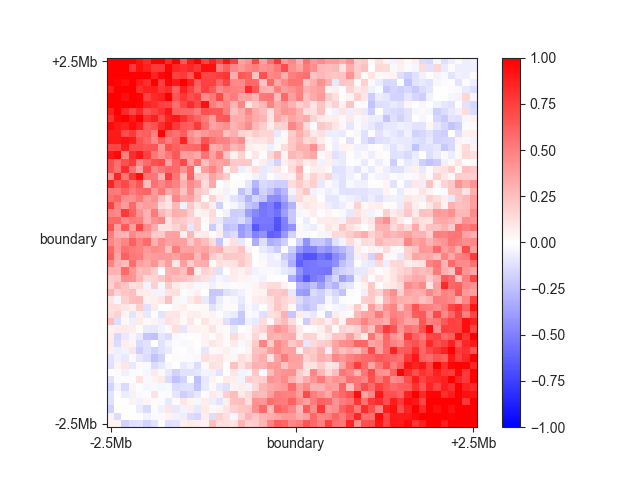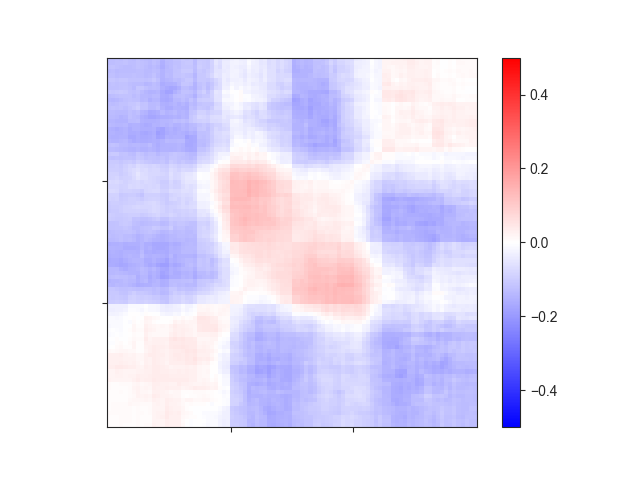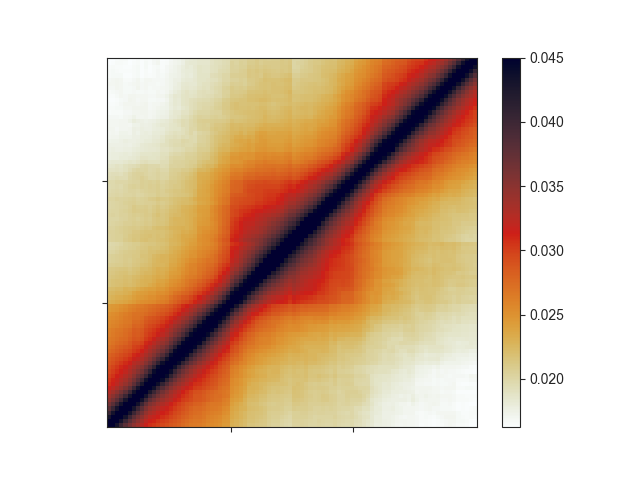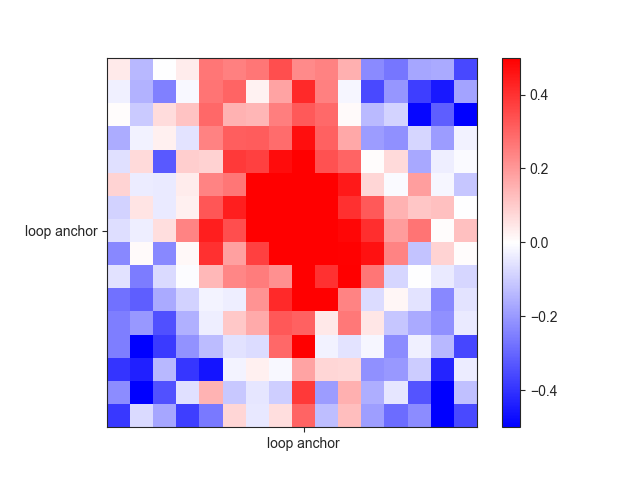# Aggregate analyses¶

To follow this tutorial, download the FAN-C example data, for example through our Keeper library. Then run the example `fanc auto` command in Example analysis to generate all the necessary files, and set up your Python session like this:

```import fanc
import fanc.plotting as fancplot

import logging
logging.basicConfig(level=logging.INFO, format="%(asctime)s %(levelname)s %(message)s")

```

If you want to try the tutorial with an equivalent Cooler file, load the Hi-C file like this instead:

```hic_100kb = fanc.load("architecture/other-hic/fanc_example.mcool@100kb")
```

or like this if you want to work with a Juicer file built from the same data:

```hic_100kb = fanc.load("architecture/other-hic/fanc_example.juicer.hic@100kb")
```

Note that there may be minor differences in the results due to the “zooming” and balancing applied by the different tools.

Also have a look at the command line documentation at Hi-C aggregate analysis, which explains the different types of aggregate analyses with helpful illustrations.

Aggregate analyses can provide a general overview of the 3D genomic structure around a set of interesting genomic regions. This is achieved by aggregating, i.e. averaging, the matrices around each region to obtain a single, aggregated view of all regions at the same time.

FAN-C distinguished three kinds of aggregate analyses:

1. Fixed-size regions along the matrix diagonal, for example a window of 500kb around each active TSS in the genome
2. Variable-size regions along the matrix diagonal, for example TADs in the genome or genes from 5’ to 3’
3. Region pairs, denoting a part of the matrix off the diagonal, for example loops in the Hi-C matrix

All of these can be computed using the `AggregateMatrix` class, and dedicated functions for each analysis type.

## Fixed-size regions¶

Fixed-size analyses are the simplest aggregate analyses. All you need is a list of regions of interest, for example insulating boundaries, and they can be computed using `from_center()` like this:

```fixed_am = fanc.AggregateMatrix.from_center(hic_100kb, boundaries.regions,
window=5000000)
```

You can optionally supply a `file_name` to save the aggregate matrix and all its components to disk.

The `window` parameter is crucial here and sets the window around the center of each region that should be extracted. If the window lies outside the chromosome boundaries, the region is declared as “invalid” and not used for the aggregate matrix. Despite being named `from_center`, it is possible to use another point in each region as relative window center with `region_viewpoint`. You can set this to any of `start`, `end`, `five-prime`, and `three_prime` and the window will be centred on that part of each region. For example, use `five_prime` to aggregate the region around the TSS’s in a list of genes.

You can plot the aggregate matrix using the covenience function `aggregate_plot()`:

```ax = fancplot.aggregate_plot(fixed_am, vmin=-1, vmax=1,
labels=['-2.5Mb', 'boundary', '+2.5Mb'])
```In this case you can nicely see the boundary in the centre of the plot. By default, an aggregate analysis always uses O/E transformed matrices, as otherwise the distance decay might bias the result too much, particular for a chromosome-based normalisation strategy, as is the default in FAN-C. You can switch this off using `oe=False`, however.

## Variable-size regions¶

The command for variable-size regions such as TADs is highly similar:

```variable_am = fanc.AggregateMatrix.from_regions(hic_100kb, tads.regions)
```

By default, each region is extended by each own length on each side (`relative_extension=1.0`), which is a useful preset for TADs so that the regions are clearly visible in the centre.

We can visualise the region using `aggregate_plot()`

```ax = fancplot.aggregate_plot(variable_am, vmin=-0.5, vmax=0.5,
relative_label_locations=[0.33, 0.66])
```To generate an aggregate matrix from variable-size regions, the extracted matrices first have to be extrapolated to the same size. This is done internally using `resize()` from the `skimage.transform` module. You can determine the type of interpolation using the `interpolation` argument, which is an integer: 0: Nearest-neighbor (default), 1: Bi-linear, 2: Bi-quadratic, 3: Bi-cubic, 4: Bi-quartic, 5: Bi-quintic. You can also turn off `antialiasing` in case you feel that this is a source of bias.

For TADs or other regions along the diagonal specifically, you can also `rescale` the aggregate matrix, which applies an artificial exponential decay to the matrix, making it resemble a Hi-C matrix:

```rescale_am = fanc.AggregateMatrix.from_regions(hic_100kb, tads.regions,
rescale=True, log=False)
```
```ax = fancplot.aggregate_plot(rescale_am, vmax=0.045,
relative_label_locations=[0.33, 0.66],
colormap='germany')
```## Region pairs¶

So far, we have only aggregated regions along the diagonal. `from_region_pairs()` allows us to aggregate arbitrary region pairs, albeit without extrapolation of differently-sized regions - only fixed window sizes are supported. You can either supply a `window`, as with fixed-size regions along the diagonal, or a number of `pixels`/bins, which is set to `16` by default.

Here is an example for loops:

```loops_am = fanc.AggregateMatrix.from_center_pairs(hic_rao, loops.region_pairs())
```
```ax = fancplot.aggregate_plot(loops_am, vmin=-0.5, vmax=0.5,
relative_label_locations=[0.5],
labels=['loop anchor'])
```## Useful functions¶

If you don’t just want to plot the aggregate matrix, but work with its values, you can access it as a `numpy` array via `matrix()`:

```m = fixed_am.matrix()
type(m)  # numpy.ndarray
```

All aggregate functions discussed above have a `keep_components` parameter, which is `True` by default. This way, all the matrix that have been extracted from regions or region pairs are stored inside the aggregate matrix object. You can access them via `components()`:

```for component_matrix in fixed_am.components():
shape = component_matrix.shape  # 51, 51
# ...
```

These individual matrices can be very useful to debug a troublesome aggregate plot, because often it is unusual outlier matrices that have a large effect on the plot. They are simply `numpy` arrays, which you can plot with `imshow` from `matplotlib`, for example.